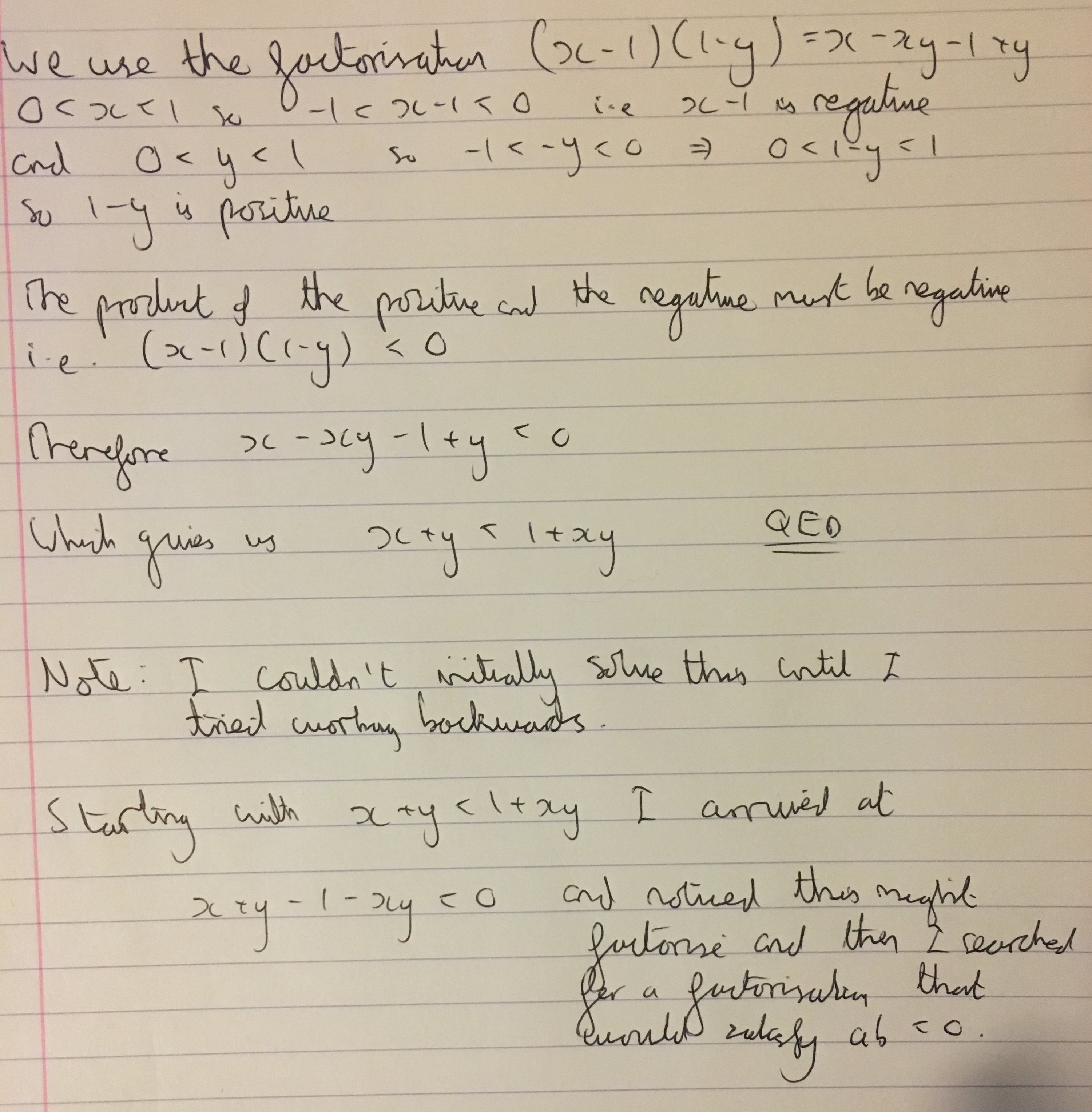#### You may also like### Circles Ad Infinitum

A circle is inscribed in an equilateral triangle. Smaller circles touch it and the sides of the triangle, the process continuing indefinitely. What is the sum of the areas of all the circles?### Areas and Ratios

Do you have enough information to work out the area of the shaded quadrilateral?### Climbing Powers

$2\wedge 3\wedge 4$ could be $(2^3)^4$ or $2^{(3^4)}$. Does it make any difference? For both definitions, which is bigger: $r\wedge r\wedge r\wedge r\dots$ where the powers of $r$ go on for ever, or $(r^r)^r$, where $r$ is $\sqrt{2}$?

# Unit Interval

##### Age 16 to 18 ShortChallenge Level

We received a lot of solutions to this problem, so thank you to Amrit and Adithya from Hymers College, Minhaj from St Ivo School, Sasi from Kimberley STEM College, Ramana from St Joseph Secondary School in Canada, Ratan from Kansas State University in the United States of America, Julian from The British School Manila in the Phillipines, Daniel from Eastbury Community School, Matthew from Highcliffe School and Ryuzaki for sending us your solutions.

There were a variety of ways to solve this problem. Here is Sasi's solution:

\begin{align} x+y &< 1+xy\\ xy-x-y+1 &> 0\\ x(y-1)-y+1 &> 0\qquad\qquad \text{Taking x out as a factor}\\ x(y-1)-(y-1) &> 0\qquad\qquad \text{Taking -1 out as a factor}\\ (x-1)(y-1) &> 0\qquad\qquad \text{Taking (y-1) out as a factor} \end{align}
If $0 < x < 1$ and $0 < y < 1$, then both $(x-1)$ and $(y-1)$ are less than $0$, so $(x-1)$ and $(y-1)$ are less than $0$, so $(x-1)(y-1)$ is greater than $0$. So the inequality is satisfied when $0 < x < 1$ and $0 < y < 1$.

Matthew used a similar solution but found his factorisation in a different way, explaining his thought process:

The initial equation is a clue to help us solve the problem. By rearranging the equation and taking all of the terms onto one side, we have an expression that is less than (or greater than) zero. Furthermore, if we have two terms that are greater than zero all we have to do is somehow make one of them less than zero and find the product, which we know will also be less than zero. This will then match what we have done to the equation and then we can try and factorise.

As $x$ and $y$ are both between $1$ and $0$, I chose to subtract $1$ from $x$, making $(x-1)$ negative. I know the equation has a $1$ and an $xy$ in it so I need an expression with a $1$ and a $y$ in it. Also, I need an expression that is greater than zero, as explained above. Therefore, I chose $(1-y)$. Now by expanding $(x-1)(1-y)$ the rest of the solution is obvious, and is described in the image below:Ryuzaki built their solution up from the initial conditions for $x$ and $y$:

We have that
\begin{align} 0<1-x&<1\qquad\qquad &\\ y(1-x)&<1-x\qquad\qquad &\text{Since 0 < y < 1}\\ y - xy&<1-x\qquad\qquad &\text{Expanding}\\ y-xy+x&<1\qquad\qquad &\text{Rearranging}\\ x+y&<1+xy\qquad\qquad &\text{Rearranging further} \end{align}

Minhaj used a very different method. He wrote $x$ and $y$ as rational numbers and then proved the result by contradiction:

We can rearrange the inequality above to $x+y-xy < 1$. So if we prove that the difference between the sum of $x$ and $y$ and the product of $x$ and $y$ is less than $1$, we have proved the original statement.

If we let $x = \frac{m}{n}$ where $n>m$ and let $y = \frac{p}{q}$ where $q>p$ and where $m$, $n$, $p$ and $q$ are Natural Numbers and $n$ is non zero, then $x$ and $y$ are rational numbers between $0$ and $1$ (as their denominator is greater than their numerator). Then we have that
\begin{align} x+y - xy &= \frac{m}{n} + \frac{p}{q} - \frac{mp}{nq}\\ &= \frac{mq + np - mp}{nq}\\ &= \frac{m(q-p) + np}{nq}\\ &= \frac{n[\frac{m(q-p)}{n} + p]}{nq} \end{align}
Now I will proceed to prove the original statement using proof by contradiction.
The three cases for $x+y-xy$ are $x+y-xy=1$, $x+y-xy>1$ and $x+y-xy<1$.
Now lets assume that $x+y-xy \geq 1$. Then
\begin{align} \frac{n[\frac{m(q-p)}{n} + p]}{nq} &\geq 1\\ \frac{m(q-p)/n + p}{q} &\geq 1\qquad\qquad\text{Cancelling n}\\ \frac{m(q-p)}{n} + p &\geq q\\ m(q-p) &\geq n(q-p)\\ \end{align}
What we have just discovered is that if $x+y - xy \geq 1$ then $m \geq n$ but this cannot be the case as we already said that $x = \frac{m}{n}$ where $n > m$ ($m$ cannot be greater than or equal to $n$ because then $x$ wouldnt be between $0$ and $1$). Hence we have proven that $x+y - xy$ cannot be equal to or greater than $1$. Therefore the only other case left is $x+y-xy <1$.
Therefore $x+y<1+xy$.